Odd cycles and low oddness

 Importance: Medium ✭✭
 Author(s):
 Subject: Graph Theory
 Keywords:
 Posted by: Gagik on: January 15th, 2010
Conjecture   If in a bridgeless cubic graphthe cycles of any-factor are odd, then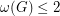, wheredenotes the oddness of the graph, that is, the minimum number of odd cycles in a-factor of.

This conjecture is false.

For oddand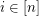, letbe the graph obtained by deleting an edge, say, from the Petersen graph. Defineto be the graph obtained by joining vertexand vertexwith an edge (with subscripts reduced modulo). For each, the setis an edge cut. Hence, in any 2-factor of, either none of the edges of the form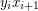are contained in a cycle, or all of them are contained in the same cycle.

Case 1: If none of the edges described above are contained in a cycle of a 2-factor of, then this 2-factor contains a 2-factor offor each. These 2-factors are also 2-factors of the graphs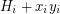, that is, each is a 2-factor of the Petersen graph. Each 2-factor of the Petersen graph consists of two cycles of 5 vertices, hence, any such 2-factor ofcontains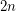cycles of odd length.

Case 2: See the comment below.

This conecture is false.

Case 2: If all of the edges of the formare contained in the same cycle in a 2-factor of, then replacing the edgeswith the edgesconverts this 2-factor ofinto a 2-factor ofdisjoint copies of the Petersen graph. Hence, when restricted to each, the 2-factor ofconsists of a cycle with 5 vertices and a-path containing a total of 5 vertices. These paths must be joined together through the edges of the formcreating a cycle of length 5n. Hence, in this case the 2-factor ofcontainscycles of length 5 and one cycle of length 5n (which is odd).

Now,is a bridgeless cubic graph whose 2-factors contain only odd cycles, but no 2-factor ofcontains fewer than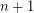cycles.

what is oddness

The notion of oddness of a graph requires explanation.

Let be a bridgeless cubic

Letbe a bridgeless cubic graph. The oddness of a 2-factoris the number of odd circuits of. The oddness ofis the smallest oddness over all 2-factors. For example, a 3-edge-colorable cubic graph has oddness zero and the Petersen graph has oddness two.